• 对抗损失函数
千次阅读
2020-05-20 20:31:31

GAN最不好理解的就是Loss函数的定义和训练过程，这里用一段代码来辅助理解，就能明白到底是怎么回事。其实GAN的损失函数并没有特殊之处，就是常用的binary_crossentropy，关键在于训练过程中存在两个神经网络和两个损失函数。

np.random.seed(42)
tf.random.set_seed(42)

codings_size = 30

generator = keras.models.Sequential([
keras.layers.Dense(100, activation="selu", input_shape=[codings_size]),
keras.layers.Dense(150, activation="selu"),
keras.layers.Dense(28 * 28, activation="sigmoid"),
keras.layers.Reshape([28, 28])
])
discriminator = keras.models.Sequential([
keras.layers.Flatten(input_shape=[28, 28]),
keras.layers.Dense(150, activation="selu"),
keras.layers.Dense(100, activation="selu"),
keras.layers.Dense(1, activation="sigmoid")
])
gan = keras.models.Sequential([generator, discriminator])

discriminator.compile(loss="binary_crossentropy", optimizer="rmsprop")
discriminator.trainable = False
gan.compile(loss="binary_crossentropy", optimizer="rmsprop")

batch_size = 32
dataset = tf.data.Dataset.from_tensor_slices(X_train).shuffle(1000)
dataset = dataset.batch(batch_size, drop_remainder=True).prefetch(1)


这里generator并不用compile，因为gan网络已经compile了。具体原因见下文。

训练过程的代码如下

def train_gan(gan, dataset, batch_size, codings_size, n_epochs=50):
generator, discriminator = gan.layers
for epoch in range(n_epochs):
print("Epoch {}/{}".format(epoch + 1, n_epochs))              # not shown in the book
for X_batch in dataset:
# phase 1 - training the discriminator
noise = tf.random.normal(shape=[batch_size, codings_size])
generated_images = generator(noise)
X_fake_and_real = tf.concat([generated_images, X_batch], axis=0)
y1 = tf.constant([[0.]] * batch_size + [[1.]] * batch_size)
discriminator.trainable = True
discriminator.train_on_batch(X_fake_and_real, y1)
# phase 2 - training the generator
noise = tf.random.normal(shape=[batch_size, codings_size])
y2 = tf.constant([[1.]] * batch_size)
discriminator.trainable = False
gan.train_on_batch(noise, y2)
plot_multiple_images(generated_images, 8)                     # not shown
plt.show()                                                    # not shown


第一阶段（discriminator训练）

# phase 1 - training the discriminator
noise = tf.random.normal(shape=[batch_size, codings_size])
generated_images = generator(noise)
X_fake_and_real = tf.concat([generated_images, X_batch], axis=0)
y1 = tf.constant([[0.]] * batch_size + [[1.]] * batch_size)
discriminator.trainable = True
discriminator.train_on_batch(X_fake_and_real, y1)


这个阶段首先生成数量相同的真实图片和假图片，concat在一起，即X_fake_and_real = tf.concat([generated_images, X_batch], axis=0)。然后是label，真图片的label是1，假图片的label是0。

然后是迅速阶段，首先将discrinimator设置为可训练，discriminator.trainable = True，然后开始阶段。第一个阶段的训练过程只训练discriminator，discriminator.train_on_batch(X_fake_and_real, y1)，而不是整个GAN网络gan

第二阶段（generator训练）

# phase 2 - training the generator
noise = tf.random.normal(shape=[batch_size, codings_size])
y2 = tf.constant([[1.]] * batch_size)
discriminator.trainable = False
gan.train_on_batch(noise, y2)


在第二阶段首先生成假图片，但是不再生成真图片。把假图片的label全部设置为1，并把discriminator的权重冻结，即discriminator.trainable = False。这一步很关键，应该这么理解：

前面第一阶段的是discriminator的训练，使真图片的预测值尽量接近1，假图片的预测值尽量接近0，以此来达到优化损失函数的目的。现在将discrinimator的权重冻结，网络中输入假图片，并故意把label设置为1。

注意，在整个gan网络中，从上向下的顺序是先通过geneartor，再通过discriminator，即gan = keras.models.Sequential([generator, discriminator])。第二个阶段将discrinimator冻结，并训练网络gan.train_on_batch(noise, y2)。如果generator生成的图片足够真实，经过discrinimator后label会尽可能接近1。由于故意把y2的label设置为1，所以如果genrator生成的图片足够真实，此时generator训练已经达到最优状态，不会大幅度更新权重；如果genrator生成的图片不够真实，经过discriminator之后，预测值会接近0，由于y2的label是1，相当于预测值不准确，这时候gan网络的损失函数较大，generator会通过更新generator的权重来降低损失函数。

之后，重新回到第一阶段训练discriminator，然后第二阶段训练generator。假设整个GAN网络达到理想状态，这时候generator产生的假图片，经过discriminator之后，预测值应该是0.5。假如这个值小于0.5，证明generator不是特别准确，在第二阶段训练过程中，generator的权重会被继续更新。假如这个值大于0.5，证明discriminator不是特别准确，在第一阶段训练中，discriminator的权征会被继续更新。

简单说，对于一张generator生成的假图片，discriminator会尽量把预测值拉下拉，generator会尽量把预测值往上扯，类似一个拔河的过程，最后达到均衡状态，例如0.6, 0.4, 0.55, 0.45, 0.51, 0.49, 0.50。神经网络 tensorflow 机器学习
更多相关内容
• ## 生成对抗网络损失函数的理解

万次阅读 多人点赞 2018-12-12 17:30:08
tf.nn.sigmoid_entropy_with_logits(labels,logits) 对抗网络中生成器的目的是尽可能使生成样本分布拟合真实样本分布。 鉴别的目的是尽可能鉴别输入样本来自于真实的还是生成的。 ...

## GAN损失函数

• 对抗网络中生成器的目的是尽可能使生成样本分布拟合真实样本分布。
• 鉴别器目的是尽可能鉴别输入样本来自于真实的还是生成的。
• 大家都知道GAN的优化目标函数如下：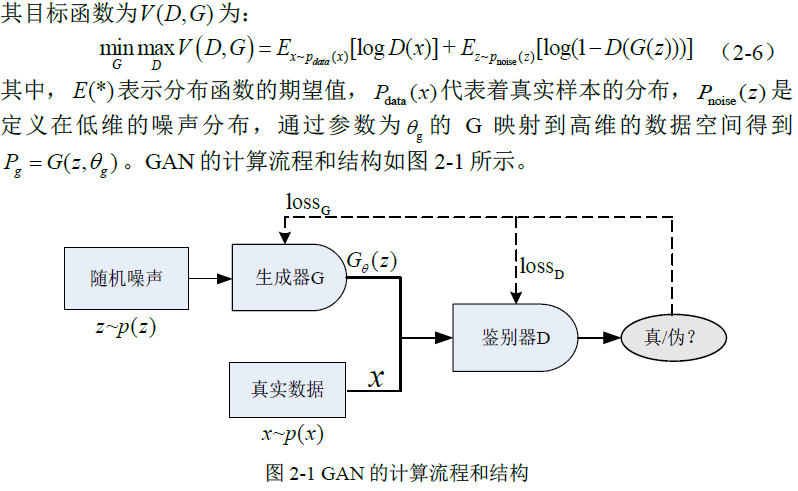• 但其参数到底是如何优化的呢？答案是交替迭代优化；如下图所示：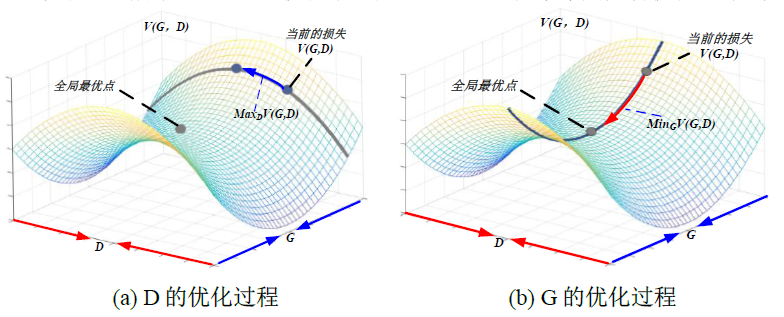• 图(a)：固定G参数不变，优化D的参数，即最大化 m a x V ( D , G ) maxV(D,G) 等价于 m i n [ − V ( D , G ) ] min[-V(D,G)] 。因此，D的损失函数等价如下：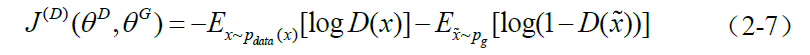• 鉴别器认为来自真实数据样本的标签为1而来自生成样本的标签为0。因此，其优化过程是类似Sigmoid 的二分类，即sigmoid的交叉熵。
• Tensorflow中的交叉熵是用tf.nn.sigmoid_entropy_with_logits(logits,labels)表示。
• 查看TF的sigmoid交叉熵API可帮助理解：
• x = logits表示鉴别器输出特征, z = labels表示对应的标签；则交叉熵表示为 z ∗ − l o g ( s i g m o i d ( x ) ) + ( 1 − z ) ∗ − l o g ( 1 − s i g m o i d ( x ) ) z * -log(sigmoid(x)) + (1 - z) * -log(1 - sigmoid(x)) .
• 推导如下：
z ∗ − l o g ( s i g m o i d ( x ) ) + ( 1 − z ) ∗ − l o g ( 1 − s i g m o i d ( x ) ) z * -log(sigmoid(x)) + (1 - z) * -log(1 - sigmoid(x))
= z ∗ − l o g ( 1 / ( 1 + e − x ) ) + ( 1 − z ) ∗ − l o g ( e − x / ( 1 + e − x ) ) = z * -log(1 / (1 +e^{-x})) + (1 - z) * -log(e^{-x} / (1 +e^{-x}))
= z ∗ l o g ( 1 + e − x ) + ( 1 − z ) ∗ ( − l o g ( e − x ) + l o g ( 1 + e − x ) ) = z * log(1 + e^{-x}) + (1 - z) * (-log(e^{-x}) + log(1 + e^{-x}))
= z ∗ l o g ( 1 + e − x ) + ( 1 − z ) ∗ ( x + l o g ( 1 + e − x ) ) = z * log(1 + e^{-x}) + (1 - z) * (x + log(1 + e^{-x}))
= ( 1 − z ) ∗ x + l o g ( 1 + e − x ) = (1 - z) * x + log(1 + e^{-x})
= x − x ∗ z + l o g ( 1 + e − x ) = x - x * z + log(1 + e^{-x})
x<0,可进一步化简为：
= l o g ( e x ) − x ∗ z + l o g ( 1 + e − x ) = log(e^x) - x * z + log(1 + e^{-x})
= − x ∗ z + l o g ( 1 + e x ) = - x * z + log(1 + e^{x})
• The logistic loss formula from above is x - x * z + log(1 + exp(-x))
• For x < 0, a more numerically stable formula is -x * z + log(1 + exp(x))
• Note that these two expressions can be combined into the following:max(x, 0) - x * z + log(1 + exp(-abs(x)))
• z=1时，真实样本对应的损失为： − l o g ( s i g m o i d ( x ) ) = l o g ( e − x + 1 ) = l o g ( e x + 1 ) − x -log(sigmoid(x))=log(e^{-x}+1)=log(e^x+1)-x .
• z=0时，生成样本对应的损失为： − l o g ( 1 − s i g m o i d ( x ) ) = x + l o g ( e − x + 1 ) = l o g ( e x + 1 ) -log(1-sigmoid(x))=x+log(e^{-x}+1)=log(e^x+1) .其中 s o f t p l u s ( x ) = l o g e ( 1 + e x ) softplus(x)=log_e(1+e^x) .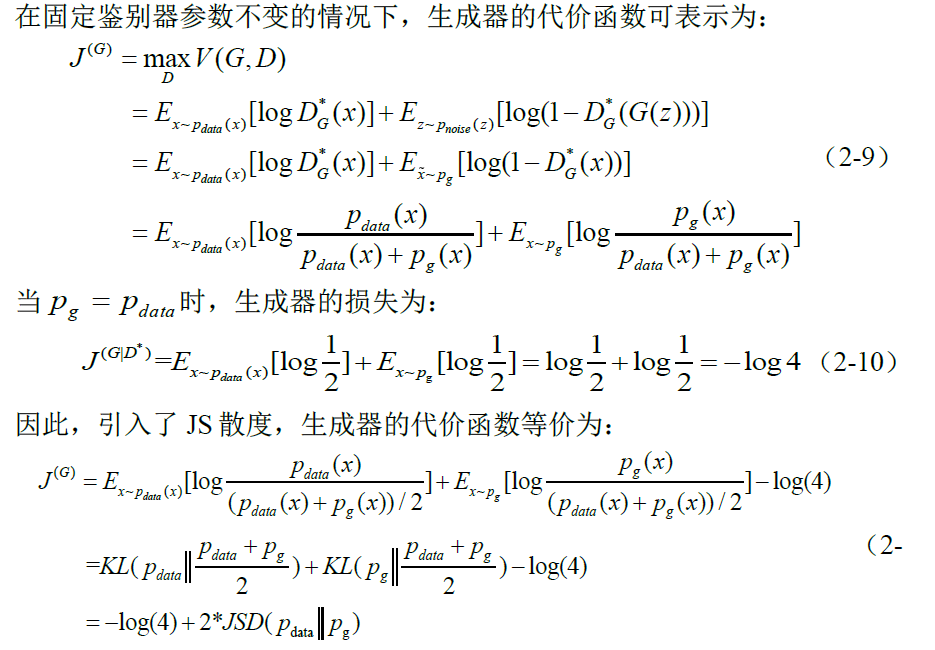• 由于JS散度具有非负性，当两者分布相等时，其散度为零。因此，D(x)训练得越好，G(z)就越接近最优，则生成器的损失越接近于生成样本分布和真实样本分布的JS 散度。
• GAN网络算法流程如下表：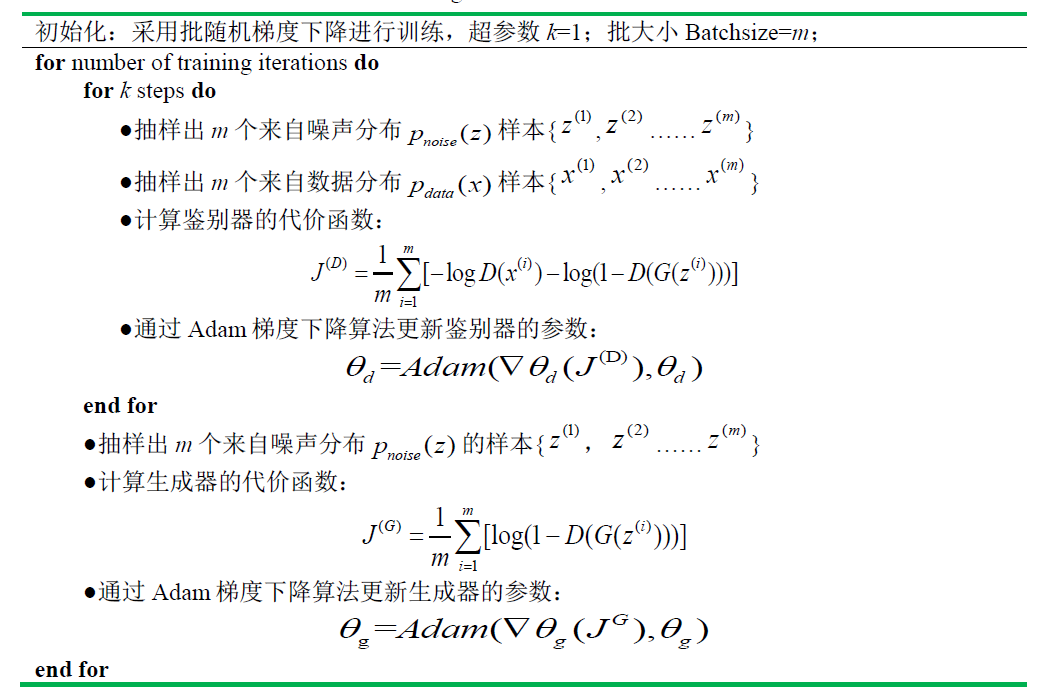• 实际上，式（2-6）可能并没有提供足够的梯度来更新G 的参数。训练初期， 由于G 没有得到较好的训练，生成样本很差，D 会以高置信度的概率来拒绝初期生成的样本，导致log(1−D(G(z)))达到饱和，无法提供足够的梯度来更新 G。于是，采用最大化log(D(G(z)))来代替最小化log(1−D(G(z)))更新 G的参数。
• tensorflow框架下的GAN的损失代码如下：
# the first term of discriminator loss of real sample:-log[D(x)]
d_loss_real = tf.reduce_mean(tf.nn.sigmoid_entropy_with_logits(logits=D_real_logits,labels=tf.ones_like(D));
# the second term of discriminator loss of fake sample:-log[1-D(G(z))]
d_loss_fake = tf.reduce_mean(tf.nn.sigmoid_entropy_with_logits(logits=D_fake_logits,labels=tf.zeros_like(D));
# D_fake_logits是鉴别器对生成器生成样本提取的特征 D(G(z))
d_loss = d_loss_real + d_loss_fake ;
# -log[D(G(z))]
g_loss = tf.reduce_mean(tf.nn.sigmoid_entropy_with_logits(logits=D_fake_logits,labels=tf.ones_like(D));

• D表示对应维度大小为batchsize的标签
展开全文GAN损失函数 sigmoid交叉熵 生成对抗网络
• 原论文中，关于生成器和判别器的损失函数是写成以下形式： 首先，第一个式子我们不看梯度符号的话即为判别器的损失函数，logD(xi)为判别器将真实数据判定为真实数据的概率，log(1-D(G(zi)))为判别器将生成器生成的...

生成式对抗网络即GAN由生成器和判别器组成。原论文中，关于生成器和判别器的损失函数是写成以下形式：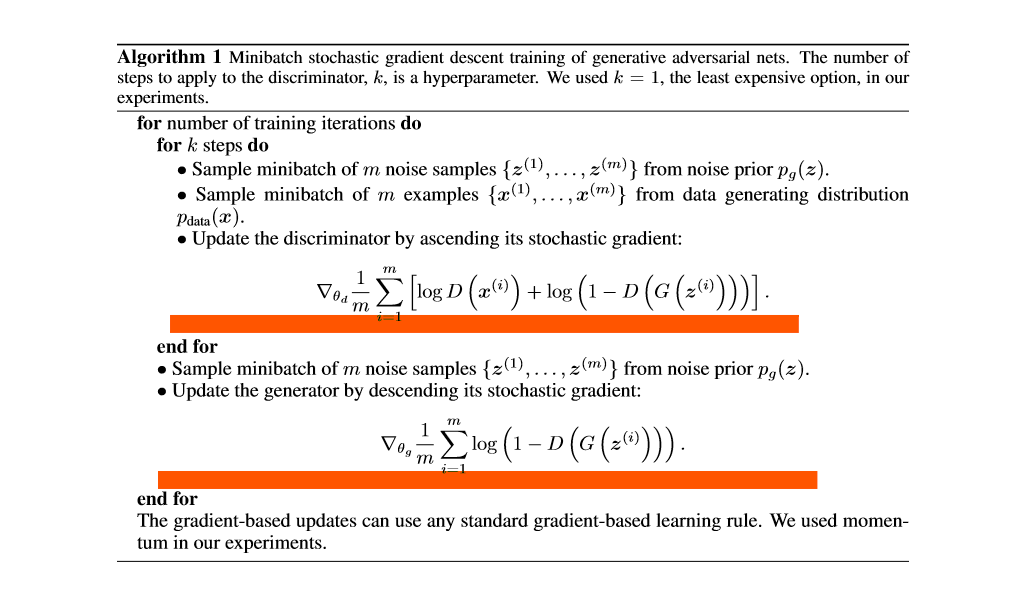首先，第一个式子我们不看梯度符号的话即为判别器的损失函数，logD(xi)为判别器将真实数据判定为真实数据的概率，log(1-D(G(zi)))为判别器将生成器生成的虚假数据判定为真实数据的对立面即将虚假数据仍判定为虚假数据的概率。判别器就相当于警察，在鉴别真伪时，必须要保证鉴别的结果真的就是真的假的就是假的，所以判别器的总损失即为二者之和，应当最大化该损失。由于判别器（警察）鉴别真伪的能力随着训练次数的增加越来越高，生成器就要与之“对抗”，生成器就要相应地提高“造假”技术，来迷惑判别器。第二个式子为第一个式子的第二项，含义相同，只不过对于生成器应当最小化该项，生成器当然希望辨别器将虚假数据仍判定为虚假数据的概率越低越好，即将虚假数据误判定为真实数据的概率越大越好，即最大化log(D(G(zi)))损失函数。所以二者相互提高或者减小自身的损失，以不断互相对抗。
GitHub上的Deep Convolutional Generative Adversarial Networks（DCGAN）的损失函数是用nn.BCELoss()来写的，具体如下：

import torch
from torch.autograd import Variable

batch_size = 10

valid = Variable(torch.Tensor(batch_size, 1).fill_(1.0), requires_grad=False)
fake = Variable(torch.Tensor(batch_size, 1).fill_(0.0), requires_grad=False)

# dis：鉴别器
# gen_imgs：生成器生成图像
# real_imgs：真实图像
g_loss = adversarial_loss(dis(gen_imgs), valid)

real_loss = adversarial_loss(dis(real_imgs), valid)
fake_loss = adversarial_loss(dis(gen_imgs), fake)
d_loss = real_loss + fake_loss



nn.BCELoss的计算公式这里不再赘述，可以查看官方文档，我手写一下代码中的g_lossd_loss: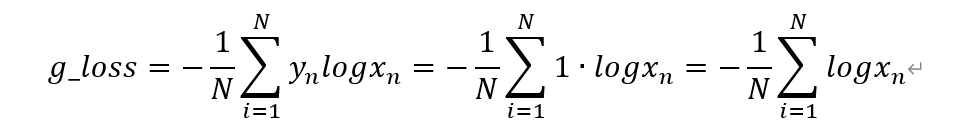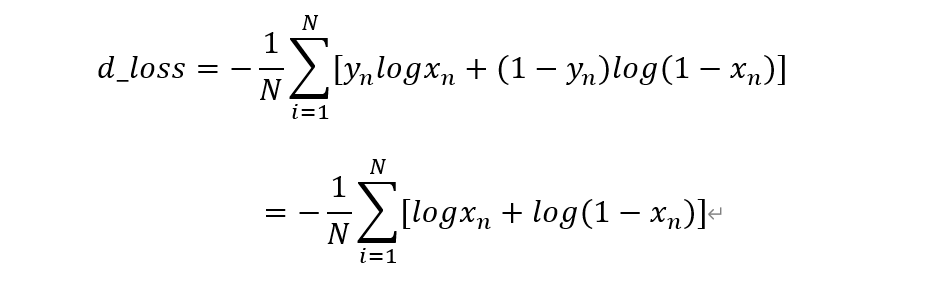torch中都是最小化损失函数，所以d_loss能理解，而g_loss只不过对原论文中的写法换了一种表述，即最大化D(G(Z))的概率：使得鉴别器将生成器生成的图像鉴别为真的概率越大越好。我用pytorch搭建了一个简易的GAN，没用卷积层，只是单纯的全连接层，利用mnist图像作为真实数据，随机生成100维的随机噪声作为生成器的输入，20次迭代的最终结果如上图，可以看出GAN多多少少能有些真实图像的大概轮廓。

展开全文• 原始的公式长这样： min⁡Gmax⁡DV(D,G)=Ex∼pdata (x)[log⁡D(x)]+Ez∼pz(z)[log⁡(1−D(G(z)))] \min _{G} \max _{D} V(D, G)=\mathbb{E}_{\boldsymbol{x} \sim p_{\text {data }}(\boldsymbol{x})}[\log D(\...

原始的公式长这样：
min ⁡ G max ⁡ D V ( D , G ) = E x ∼ p data  ( x ) [ log ⁡ D ( x ) ] + E z ∼ p z ( z ) [ log ⁡ ( 1 − D ( G ( z ) ) ) ] \min _{G} \max _{D} V(D, G)=\mathbb{E}_{\boldsymbol{x} \sim p_{\text {data }}(\boldsymbol{x})}[\log D(\boldsymbol{x})]+\mathbb{E}_{\boldsymbol{z} \sim p_{\boldsymbol{z}}(\boldsymbol{z})}[\log (1-D(G(\boldsymbol{z})))] 首先可以明确一点，这种公式肯定是从里面算到外面的，也就是可以先看这一部分： max ⁡ D V ( D , G ) = E x ∼ p data  ( x ) [ log ⁡ D ( x ) ] + E z ∼ p z ( z ) [ log ⁡ ( 1 − D ( G ( z ) ) ) ] \max _{D} V(D, G)=\mathbb{E}_{\boldsymbol{x} \sim p_{\text {data }}(\boldsymbol{x})}[\log D(\boldsymbol{x})]+\mathbb{E}_{\boldsymbol{z} \sim p_{\boldsymbol{z}}(\boldsymbol{z})}[\log (1-D(G(\boldsymbol{z})))] 我们知道，在每个epoch中，GAN的生成器与判别器是分别训练的，即先固定生成器 G G ，去训练判别器 D D ，那么上面这个式子实际上就是判别器的"损失函数"。继续拆分上面这个式子，可以发现主要就是加号左右两个部分。

先看左边。左边这一部分的作用是保证判别器的基础判断能力：对于 E x ∼ p data  ( x ) [ log ⁡ D ( x ) ] \mathbb{E}_{\boldsymbol{x} \sim p_{\text {data }}(\boldsymbol{x})}[\log D(\boldsymbol{x})] x \boldsymbol{x} 为从真实数据分布 p data  p_{\text {data }} 中采样得到的样本。 E x ∼ p data  ( x ) [ log ⁡ D ( x ) ] \mathbb{E}_{\boldsymbol{x} \sim p_{\text {data }}(\boldsymbol{x})}[\log D(\boldsymbol{x})] 越大，相当于意味着 D ( x ) D(\boldsymbol{x}) 越大，即判别器越能准确地将真实样本识别为真实样本；因此有 max ⁡ D \max _{D}

再看右边。右边这一部分的作用是保证判别器能够区分出虚假样本：对于 E z ∼ p z ( z ) [ log ⁡ ( 1 − D ( G ( z ) ) ) ] \mathbb{E}_{\boldsymbol{z} \sim p_{\boldsymbol{z}}(\boldsymbol{z})}[\log (1-D(G(\boldsymbol{z})))] z \boldsymbol{z} 为从某一特定分布 p z p_{\boldsymbol{z}} 中得到的采样， G ( z ) G(\boldsymbol{z}) 为生成器生成的虚假样本。 E z ∼ p z ( z ) [ log ⁡ ( 1 − D ( G ( z ) ) ) ] \mathbb{E}_{\boldsymbol{z} \sim p_{\boldsymbol{z}}(\boldsymbol{z})}[\log (1-D(G(\boldsymbol{z})))] 越大，相当于意味着 D ( G ( z ) ) D(G(\boldsymbol{z})) 越小，即判别器越能够正确区分虚假样本，将其标为False；因此有 max ⁡ D \max _{D}

再来看生成器G的"损失函数"。到了训练生成器G的阶段，此时判别器D固定。如果G更强，那么判别器会进行误判，此时 D ( G ( z ) ) D(G(\boldsymbol{z})) 会变大， E z ∼ p z ( z ) [ log ⁡ ( 1 − D ( G ( z ) ) ) ] \mathbb{E}_{\boldsymbol{z} \sim p_{\boldsymbol{z}}(\boldsymbol{z})}[\log (1-D(G(\boldsymbol{z})))] 更接近于零，即整个式子的值会更小；因此有 min ⁡ G \min _{G}

展开全文• 摘要： 从本质上来说，生成对抗网络（GAN）是一种特殊的损失函数，我们来深入探索下这句话的含义。   数据科学家Jeremy Howard在fast.ai的《生成对抗网络（GAN）》课程中曾经讲过这样一句话： “从本质上来说，...交叉熵损失函数
• 深度学习常用损失函数介绍， 本文主要收集和整理了深度学习常用的损失函数，包括softmax，arcface,centerloss等常见损失函数，给出函数表达式，以及使用介绍和应用场景softmax
• 再日常模型训练中，最常见的是做回归预测或者做分类，下面分别介绍它们的常用损失函数的用法。 其他损失函数的用法是一样的。可以再这份博客里看看各类损失的含义：https://www.cnblogs.com/kk17/p/10246324.html ...
• 损失函数(Loss Function) 代价函数(Cost Function) 目标函数(Objective Function) nn.CrossEntropyLoss # 使用交叉熵损失函数nn.CrossEntropyLoss 的几个模式来对比一下结果： reduction='none' reduction='sum'...pytorch 自然语言处理
• 我们的构建的模型包含两类组件(Our objective contains two types of terms)： 对抗损失(adversarial losses)，使生成的图片在分布上更接近于目标图片； 循环一致性损失(cycle consistency losses)，防止学习到的...机器学习 人工智能
• 损失函数的计算辨别器对假数据的损失原理相同，最终达到的目标是对于所有的真实图片，输出为1；对于所有的假图片，输出为0。 生成器的目标是愚弄辨别器蒙混过关，需要达到的目标是对于生成的图片，输出为1.`d_loss_...
• 对于机器学习中常见的损失函数有：平方损失函数与交叉熵损失函数。在本文中将讲述两者含义与响应的运用区别。 2. 平方损失函数 平方损失函数较为容易理解，它直接测量机器学习模型的输出与实际结果之间的距离。...交叉熵损失函数
• 图像到图像的转换的目标是使用配准的图像对训练集来学习输入图像和输出图像之间的映射，而CycleGAN中...= 对抗损失函数 + 循环损失函数 λ代表对抗损失函数 和 循环损失函数之间的权重比 总体目标 实现 Implementation生成对抗网络 计算机视觉
• ## 常用损失函数和评价指标总结

千次阅读 多人点赞 2019-09-03 23:21:11
1. 损失函数： 2.评价指标： 2.1 回归问题： 1. MSE： 均方误差（Mean Square Error），范围 [0,+∞)[0,+∞)[0,+∞) MSE=1n∑i=1n(y^i−yi)2 M S E=\frac{1}{n} \sum_{i=1}^{n}\left(\hat{y}_{i}-y_{i}\right)^{2} ...评价指标 ROC
• ## 生成对抗网络(四)CycleGAN讲解

万次阅读 多人点赞 2020-03-07 21:11:58
生成对抗网络CycleGAN讲解 Abstract 1. Introduction 2. Related Work 原文：Unpaired Image-to-Image Translation using Cycle-Consistent Adversarial Networks 收录：2018 代码：Pytorch 这次工作则是将无配对...
• 什么是损失函数 损失函数（loss function）或代价函数（cost function）是将随机事件或其有关随机变量的取值映射为非负实数以表示该随机事件的“风险”或“损失”的函数。 神经网络以某个指标为线索寻找最优权重...神经网络 人工智能
•神经网络
• 假设我们有一个卧室图像数据集和一个在这个...这使得我们的分类器模型成为一个高维概率分布函数，它给出了来自这个大输入空间的给定输入作为卧室的概率。那么，如果我们可以从卧室图像的数据分布中学习这种高维知识...gan网络损失函数
• 1.代码 from matplotlib import pyplot as plt import numpy as np w = np.linspace(-1.15,1.15,10000) Loss = (w**8-1)**2 plt.plot(w,Loss,label='9 layers NN') plt.xlabel('w') plt.ylabel('Loss') ...python
• 1.生成器（generator）的损失函数：生成器的损失函数对抗损失和像素损失构成。 def backward_G(self): """Calculate GAN and L1 loss for the generator""" # 1.对抗损失，G(A) should fake the ...
• GAN的全称是 Generative Adversarial Networks，中文名称是生成对抗网络。原始的GAN是一种无监督学习方法，巧妙的利用“博弈”的思想来学习生成式模型。1 GAN的原理GAN的基本原理很简单，其由两个网络组成，一个是...
• 所提出的重建损失提高了GAN的性能，在不同的数据集上产生了更高质量的图像，并且可以轻松地与其他正则化损失函数（例如梯度罚分）组合以提高各种GAN的性能。 我们对不同数据集上广泛采用的DCGAN体系结构和复杂的...
•迁移学习 分类 python
• 文章目录一、什么是损失函数？二、分类任务损失1、0-1 loss2、熵与交叉熵loss3、softmax loss及其变种4、KL散度5、Hinge loss6、Exponential loss与Logistic loss三、回归任务损失1、L1 loss2、L2 loss3、L1 loss与...
• 损失函数loss总结1.What2.常见的损失函数回归任务均方误差补充分类任务交叉熵(cross entropy)3.未完待续 1.What 在机器学习中，损失函数（loss function）是用来估量模型的预测值f(x)与真实值y的不一致程度。 2.常见...机器学习 数据挖掘
•   最近在基于对比学习做实验，github有许多实现，虽然直接套用即可，但是细看之下，损失函数部分甚是疑惑，故学习并记录于此。关于对比学习的内容网络上已经有很多内容了，因此不再赘述。本文重在对InfoNCE的两种...
• tf.keras实现ESRGAN相对平均判别器RaGAN损失
• Tensorflow2.0 + Transformers 实现Bert FGM抗训练惩罚梯度损失函数前言变种实现Transformers中的word_embeddings代码修改实验效果总结 前言 之前看了很多关于NLP中应用对抗训练的文章，测试结果都很香，所以想在...自然语言处理 tensorflow python
• 文章目录 写在最前面的话：本文主要整理深度学习中的损失函数，从原理到作用，详细了解损失函数GAN loss
• 由于 PSNR 的定义（第 2.3.1 节）与像素差异高度相关，并且最小化像素损失直接最大化 PSNR，因此像素损失已成为该领域使用最广泛的损失函数。 然而，由于像素损失实际上并没有考虑图像质量（例如，感知质量、...计算机视觉...